Chapter Nine Buying and Selling Endowments u The

• Slides: 29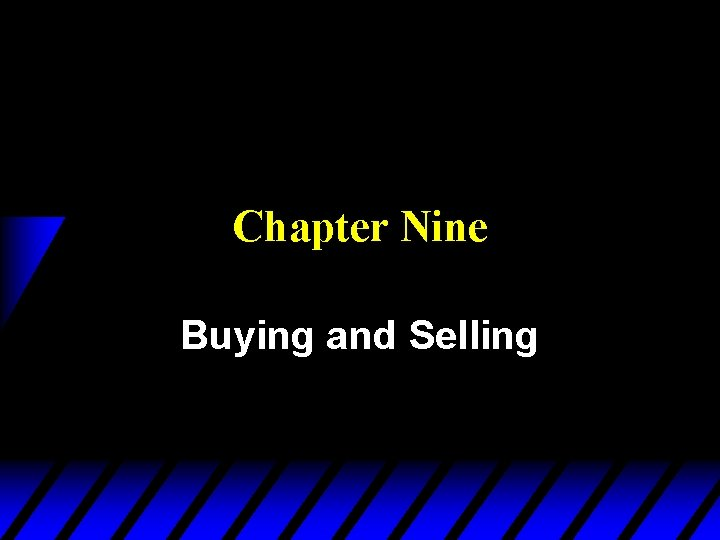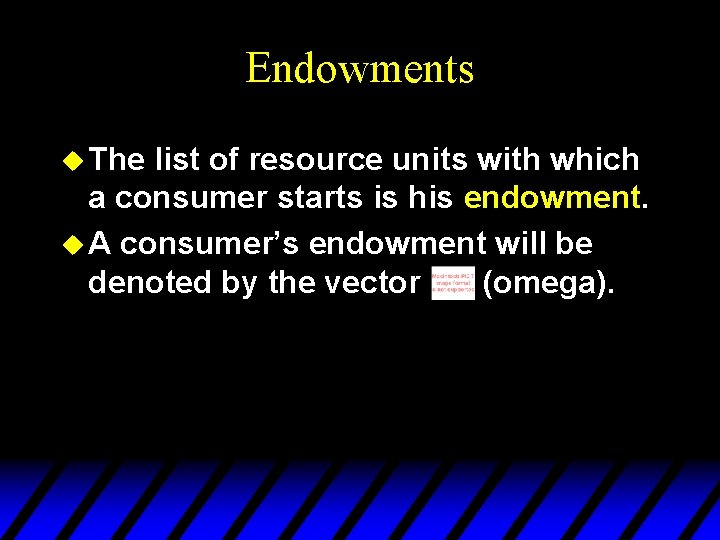Endowments u The list of resource units with which a consumer starts is his endowment. u A consumer’s endowment will be denoted by the vector (omega).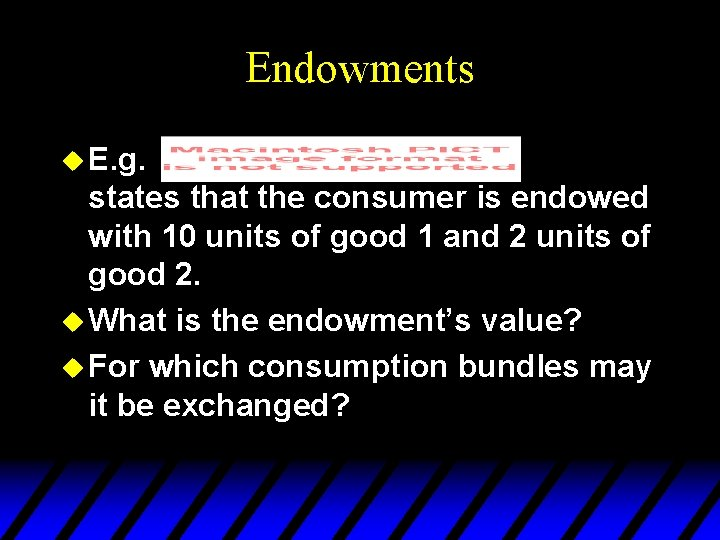Endowments u E. g. states that the consumer is endowed with 10 units of good 1 and 2 units of good 2. u What is the endowment’s value? u For which consumption bundles may it be exchanged?Endowments u p 1=2 and p 2=3 so the value of the endowment is u Q: For which consumption bundles may the endowment be exchanged? u A: For any bundle costing no more than the endowment’s value.Budget Constraints Revisited u So, given p 1 and p 2, the budget constraint for a consumer with an endowment is u The budget set is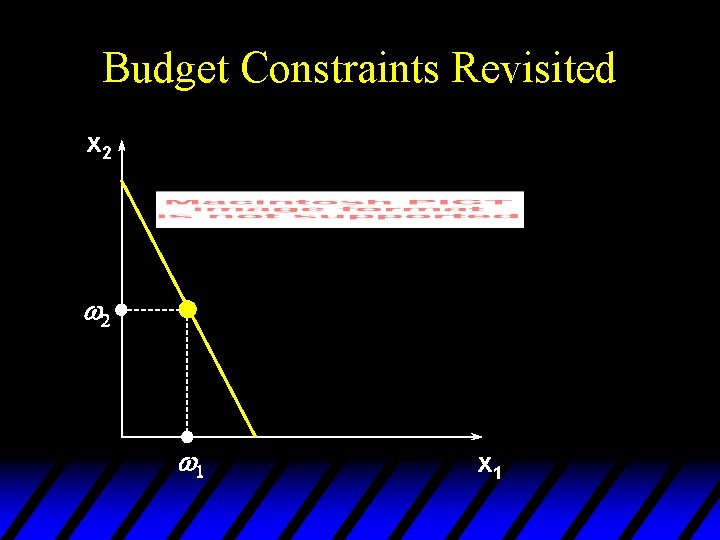Budget Constraints Revisited x 2 w 1 x 1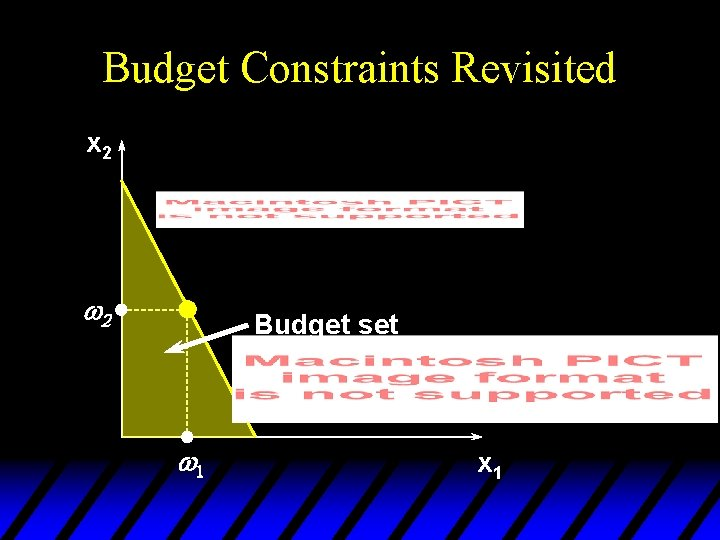Budget Constraints Revisited x 2 w 2 Budget set w 1 x 1Budget Constraints Revisited x 2 w 1 x 1Budget Constraints Revisited x 2 w 2 Budget set w 1 x 1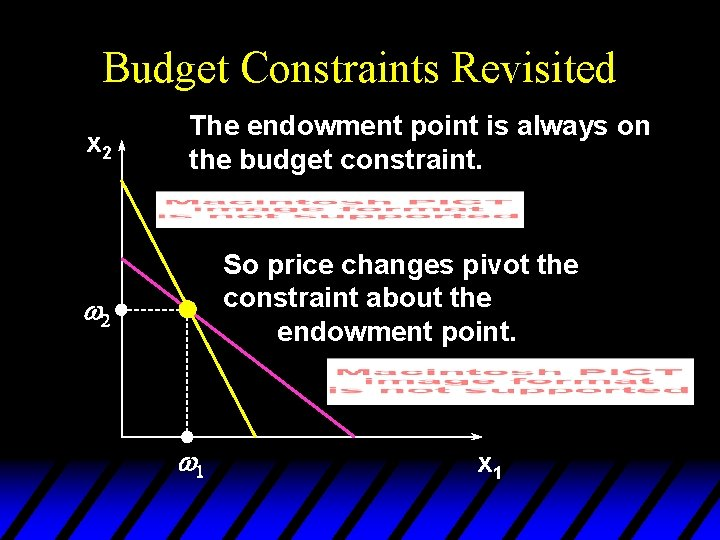Budget Constraints Revisited x 2 The endowment point is always on the budget constraint. So price changes pivot the constraint about the endowment point. w 2 w 1 x 1Budget Constraints Revisited u The constraint is u That is, the sum of the values of a consumer’s net demands is zero.Net Demands u Suppose and p 1=2, p 2=3. Then the constraint is u If the consumer demands (x 1*, x 2*) = (7, 4), then 3 good 1 units exchange for 2 good 2 units. Net demands are x 1*- w 1 = 7 -10 = -3 and x 2*- w 2 = 4 - 2 = +2.Net Demands p 1=2, p 2=3, x 1*-w 1 = -3 and x 2*-w 2 = +2 so The purchase of 2 extra good 2 units at \$3 each is funded by giving up 3 good 1 units at \$2 each.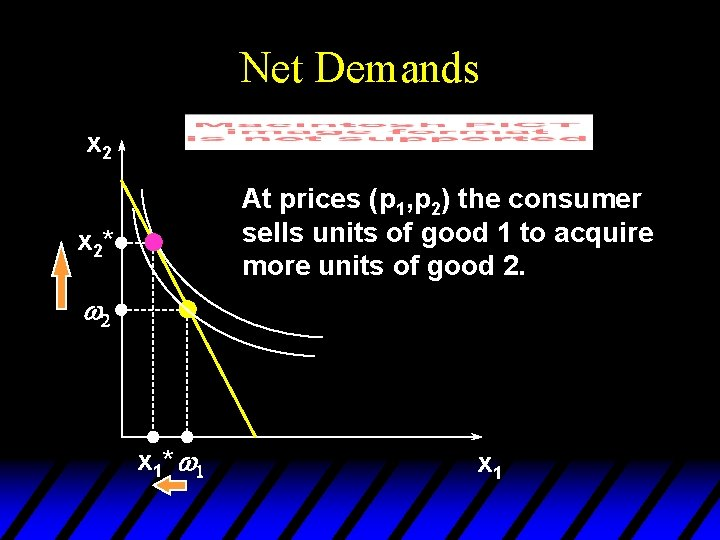Net Demands x 2 At prices (p 1, p 2) the consumer sells units of good 1 to acquire more units of good 2. x 2* w 2 x 1 * w 1 x 1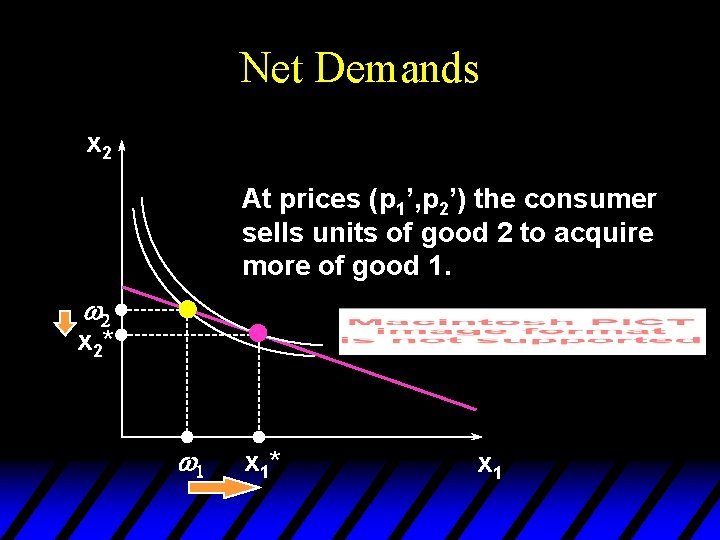Net Demands x 2 At prices (p 1’, p 2’) the consumer sells units of good 2 to acquire more of good 1. w 2 x 2* w 1 x 1* x 1Net Demands x 2 At prices (p 1”, p 2”) the consumer consumes her endowment; net demands are all zero. x 2*=w 2 x 1*=w 1 x 1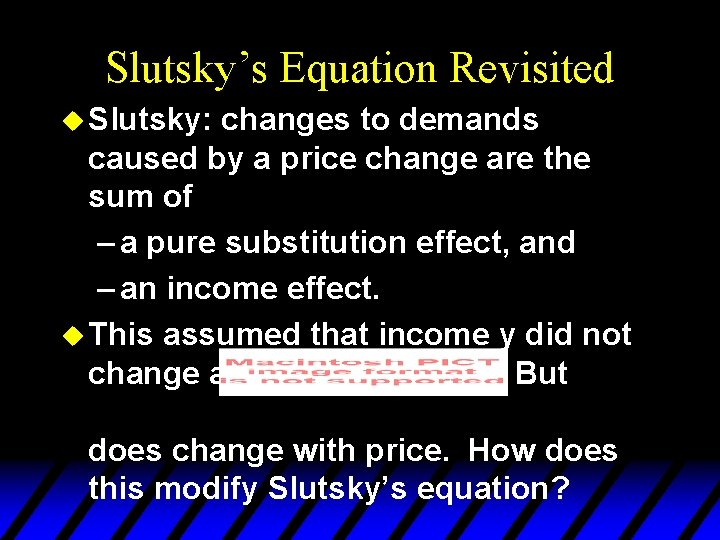Slutsky’s Equation Revisited u Slutsky: changes to demands caused by a price change are the sum of – a pure substitution effect, and – an income effect. u This assumed that income y did not change as prices changed. But does change with price. How does this modify Slutsky’s equation?Slutsky’s Equation Revisited u. A change in p 1 or p 2 changes so there will be an additional income effect, called the endowment income effect. u Slutsky’s decomposition will thus have three components – a pure substitution effect – an (ordinary) income effect, and – an endowment income effect.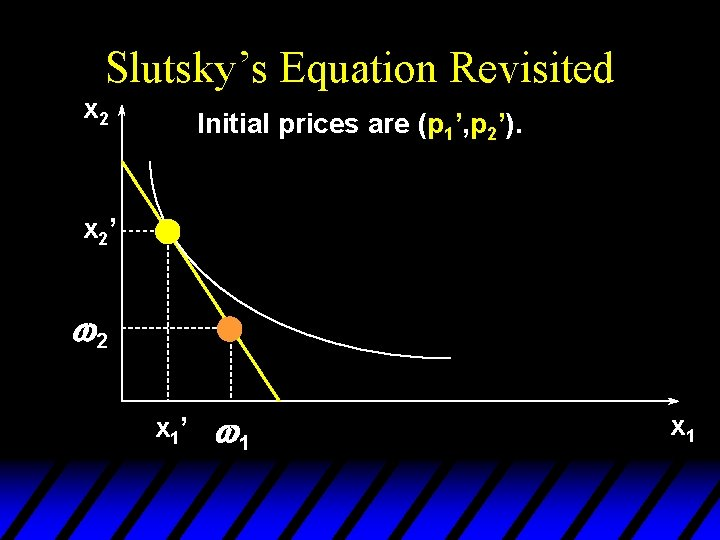Slutsky’s Equation Revisited x 2 Initial prices are (p 1’, p 2’). x 2’ w 2 x 1’ w 1 x 1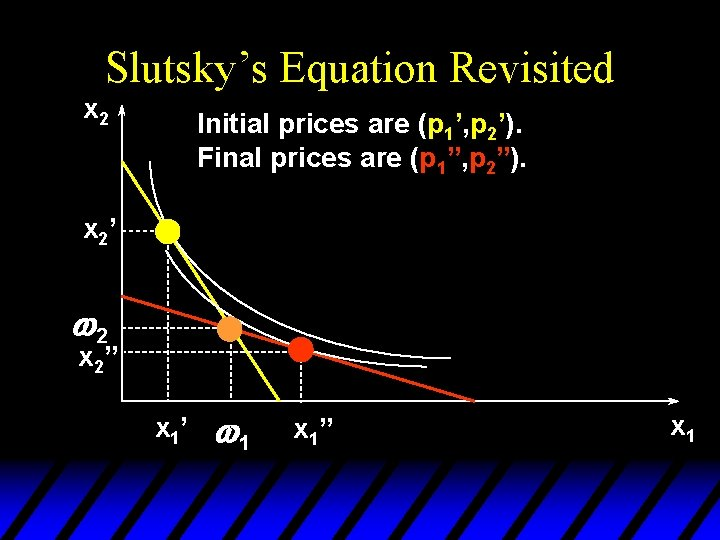Slutsky’s Equation Revisited x 2 Initial prices are (p 1’, p 2’). Final prices are (p 1”, p 2”). x 2’ w 2 x 2” x 1’ w 1 x 1” x 1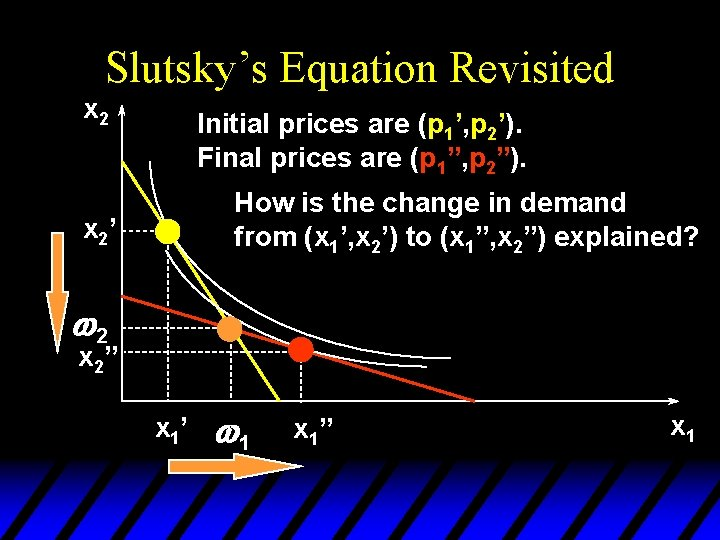Slutsky’s Equation Revisited x 2 Initial prices are (p 1’, p 2’). Final prices are (p 1”, p 2”). How is the change in demand from (x 1’, x 2’) to (x 1”, x 2”) explained? x 2’ w 2 x 2” x 1’ w 1 x 1” x 1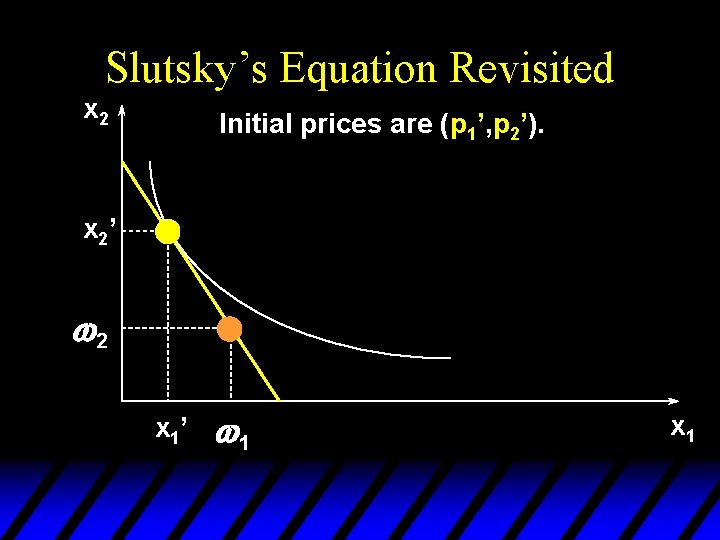Slutsky’s Equation Revisited x 2 Initial prices are (p 1’, p 2’). x 2’ w 2 x 1’ w 1 x 1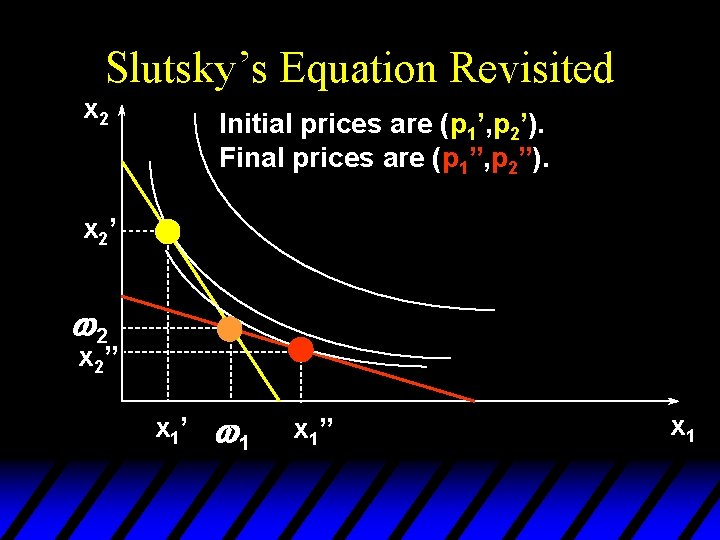Slutsky’s Equation Revisited x 2 Initial prices are (p 1’, p 2’). Final prices are (p 1”, p 2”). x 2’ w 2 x 2” x 1’ w 1 x 1” x 1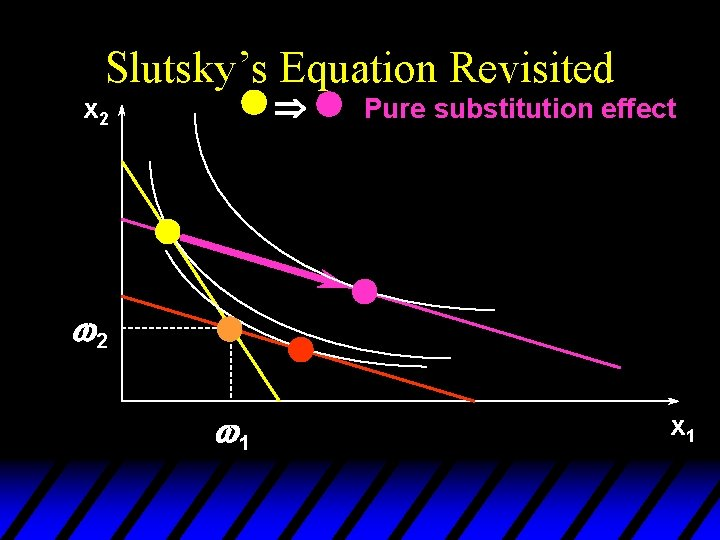Slutsky’s Equation Revisited Þ x 2 Pure substitution effect w 2 w 1 x 1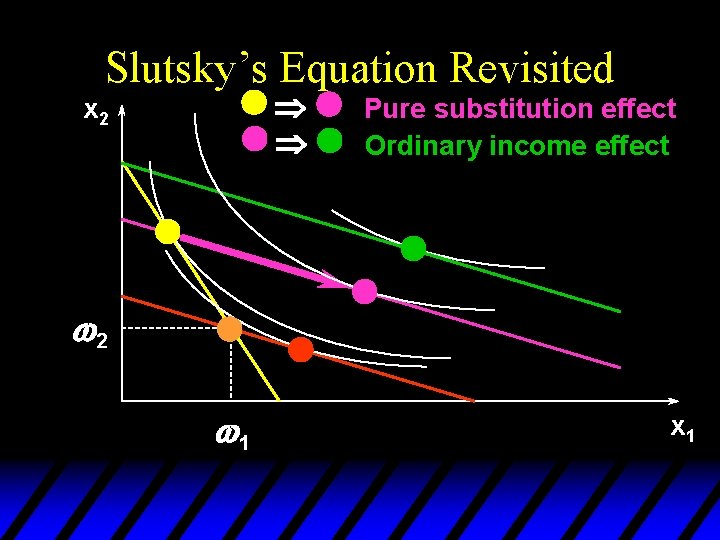Slutsky’s Equation Revisited Þ Þ x 2 Pure substitution effect Ordinary income effect w 2 w 1 x 1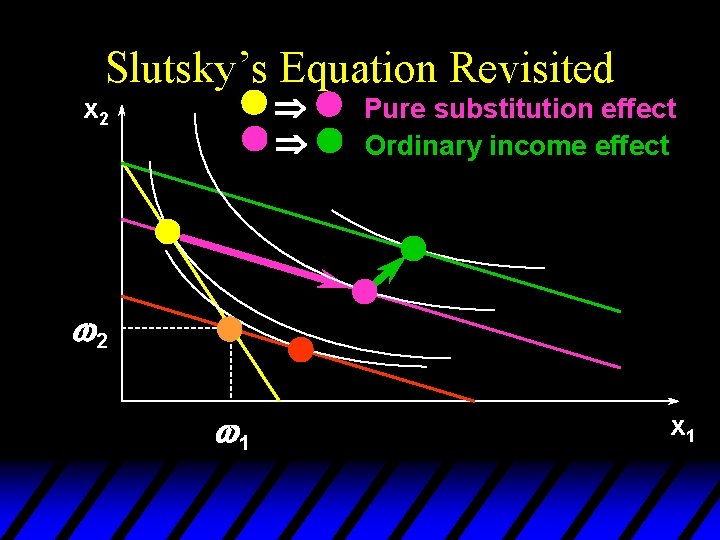Slutsky’s Equation Revisited Þ Þ x 2 Pure substitution effect Ordinary income effect w 2 w 1 x 1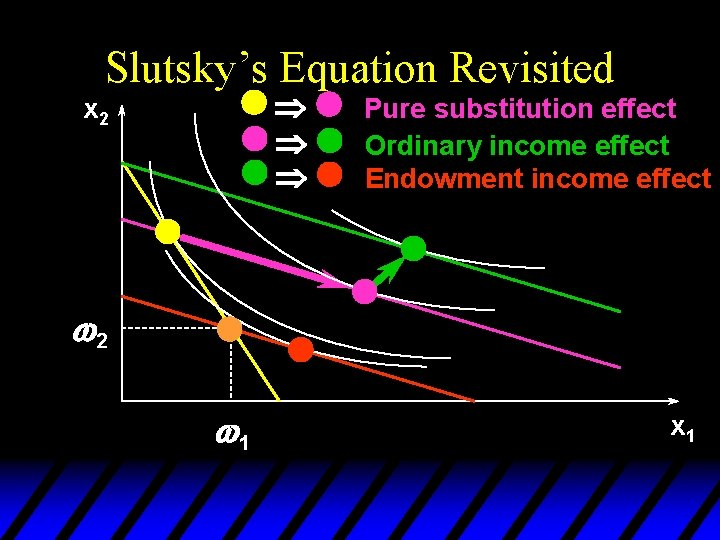Slutsky’s Equation Revisited Þ Þ Þ x 2 Pure substitution effect Ordinary income effect Endowment income effect w 2 w 1 x 1Slutsky’s Equation Revisited Þ Þ Þ x 2 Pure substitution effect Ordinary income effect Endowment income effect w 2 w 1 x 1Slutsky’s Equation Revisited Overall change in demand caused by a change in price is the sum of: (i) a pure substitution effect (ii) an ordinary income effect (iii) an endowment income effect